﻿ 渤海中部沙河街组深埋藏砂岩中优质储层成因

# 渤海中部沙河街组深埋藏砂岩中优质储层成因Characteristics and Genesis of Paleogene Deeply-Buried Sandstones Favorable Reservoirs in the Central Bohai Sea Area

Abstract: The sandstone reservoir of the Paleogene Lacustrine Shahejie Formation in Bohai is deep in depth, mainly in the middle depth of more than 3000 meters, showing the fan delta depositional system. The main rock types are lithic feldspar sandstone and feldspar lithic sandstone. The high content of feldspar is about 40.39%, and the content of quartz is low, accounting for only 20.39%. In the process of diagenesis, the compaction is generally weak, while the dissolution occupies the main position. Because of the dissolution of feldspar, the formation of authigenic minerals, especially clay minerals and authigenic quartz, plus the filling of carbonate minerals, leads to the continuous decrease of porosity. In general, the sandstone property of the study area is good, and the average porosity is 20.8%, and the permeability is 460.2 mD, which can form good reservoir. On the other hand, through scanning electron microscopy, electron microscopy, fluorescence analysis and other observations, we can understand the characteristics of porosity and permeability of different reservoirs, so as to understand the reasons for forming high-quality reservoirs.

1. 引言

2. 地质背景

3000 m，主要为微咸水湖泊环境中形成的砂岩、泥岩等碎屑岩沉积  -  。

3. 沙河街组砂岩储集性特征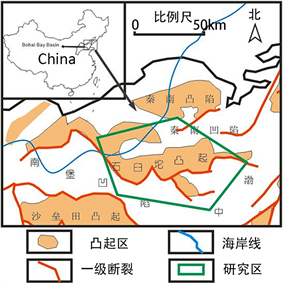Figure 1. The position structure map of the central study area in the Bohai Bay Basin (according to Lv Zhengxiang, 2015)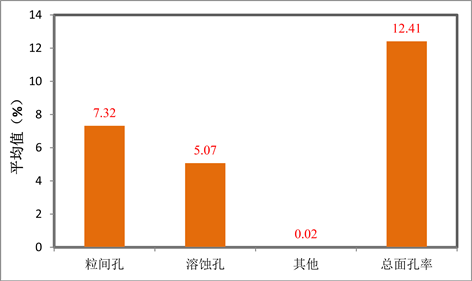Figure 2. Distribution histogram of pore type of sandstone reservoir in Shahejie formation

4. 优质储层特征

5. 优质储层发育成因

1) 沉积古环境水动力强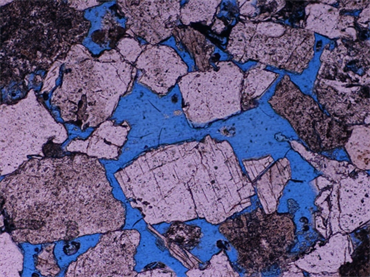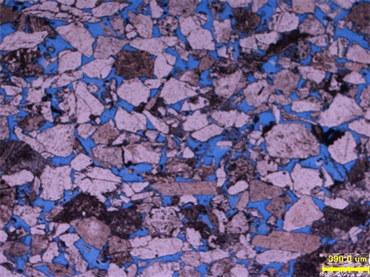BH2井，左(-)，中砂岩中粒间孔发育 BH2井，右(-)，粒间溶蚀扩大孔，颗粒呈漂浮状

Figure 3. Pore type of sandstone reservoir in Shahejie formation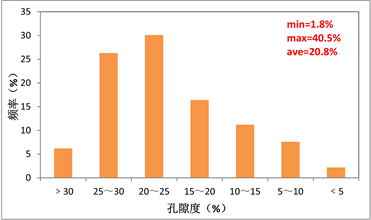Figure 4. Reservoir sandstone porosity and permeability distribution histogram of Shahejie formation

E2s的微咸水湖泊环境沉积微相表明优质储层主要在河道和河口砂坝区域发育(图6)，因为这些地区具有较强的水动力条件，促使研究区总体分布以较低杂基含量的样品为主，超过65%的样品杂基含量分布在4%以下，平均值则在3.0%左右，砂岩基本上都是低杂基含量的净砂岩(图7)。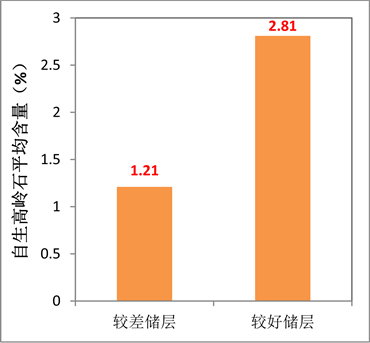Figure 5. The cement content and autogenic kaolinite difference diagram in different reservoir sandstone reservoirs of Shahejie formation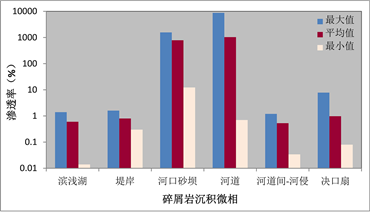Figure 6. The characteristic map of the physical distribution of different sedimentary microfacies sandstone in the Shahejie formation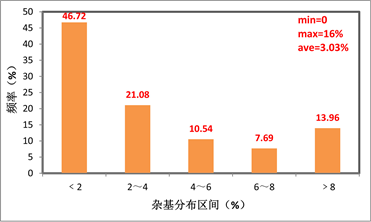Figure 7. The distribution characteristics of the complex content of the sandstone reservoirs in the Shahejie formation

2) 长石溶蚀强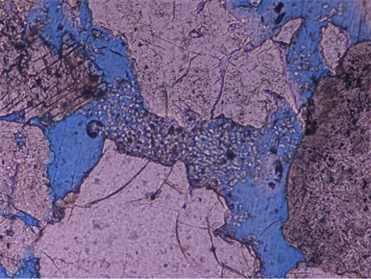Figure 8. The intergranular dissolution of BH2 well sandstone expands kaolinite filled with holes. Left (+), right (−)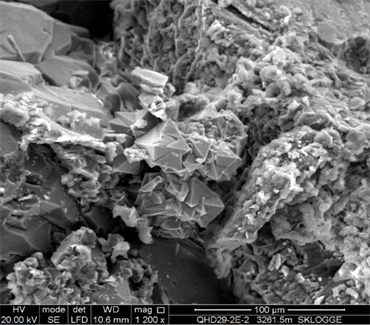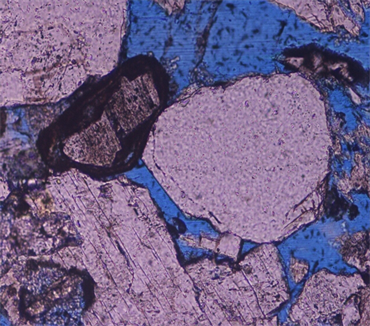BH2井砂岩中的石英加大(-) 孔隙充填石英

Figure 9. The intergranular dissolution of BH2 well sandstone expands kaolinite filled with holes. Left (+), right (−)

3) 早期烃类充注

6. 形成优质储层的长石溶蚀机理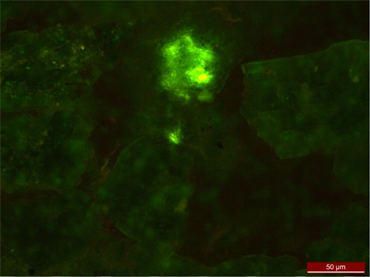Figure 10. Fluorescence characteristics in BH2 well sandstone, left (-), right (fluorescence)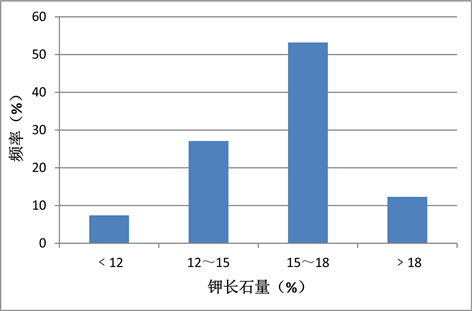Figure 11. The type of X-diffraction feldspar in clastic rock and its content distribution map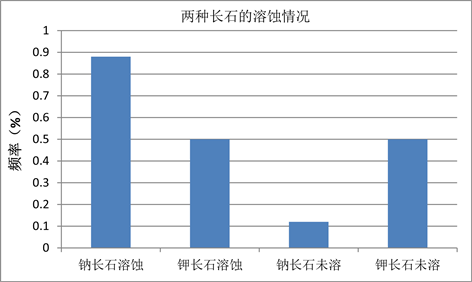Figure 12. Shahejie Formation dissolving feldspar type and its distribution frequency comparison diagram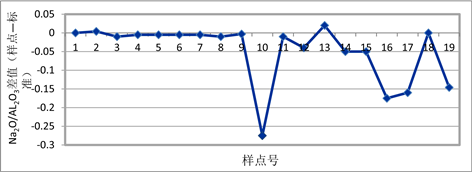Figure 13. Characteristic map of difference distribution between kaolinite and standard kaolinite Al2O3/SiO2Figure 14. Distribution map of oxygen and carbon isotopes of various carbonate minerals in Shahejie Formation

$\text{2K}\left[{\text{AlSi}}_{\text{3}}{\text{O}}_{\text{8}}\right]\left(钾长石\right)+{\text{2H}}_{\text{2}}\text{O}+{\text{CO}}_{\text{2}}⇌{\text{Al}}_{\text{2}}{\text{Si}}_{\text{2}}{\text{O}}_{\text{5}}{\left(\text{OH}\right)}_{\text{4}}\left(高岭石\right)+{\text{4SiO}}_{\text{2}}\left(石英\right)+{\text{K}}_{\text{2}}{\text{CO}}_{\text{3}}$

$\text{2Na}\left[{\text{AlSi}}_{\text{3}}{\text{O}}_{\text{8}}\right]\left(钠长石\right)+{\text{2H}}_{\text{2}}{\text{CO}}_{\text{3}}+{\text{9H}}_{\text{2}}\text{O}⇌{\text{2Na}}^{+}+{\text{2HCO}}_{3}^{-}+{\text{4H}}_{\text{4}}{\text{SiO}}_{\text{4}}+{\text{Al}}_{\text{2}}{\text{Si}}_{\text{2}}{\text{O}}_{\text{5}}{\left(\text{OH}\right)}_{\text{4}}\left(高岭石\right)$

${\text{2KAlSi}}_{\text{3}}{\text{O}}_{\text{8}}\left(钾长石\right)+{\text{2CH}}_{\text{3}}\text{COOH}+{\text{9H}}_{\text{2}}\text{O}\to {\text{Al}}_{\text{2}}{\text{Si}}_{\text{2}}{\text{O}}_{\text{5}}{\left(\text{OH}\right)}_{\text{4}}\left(高岭石\right)+{\text{2K}}^{+}+{\text{4H}}_{\text{4}}{\text{SiO}}_{\text{4}}+{\text{2CH}}_{\text{3}}{\text{COO}}^{－}$

${\text{2NaAlSi}}_{\text{3}}{\text{O}}_{\text{8}}\left(钠长石\right)+{\text{2CH}}_{\text{3}}\text{COOH}+{\text{9H}}_{\text{2}}\text{O}\to {\text{Al}}_{\text{2}}{\text{Si}}_{\text{2}}{\text{O}}_{\text{5}}{\left(\text{OH}\right)}_{\text{4}}\left(高岭石\right)+{\text{2Na}}^{+}+{\text{4H}}_{\text{4}}{\text{SiO}}_{\text{4}}+{\text{2CH}}_{\text{3}}{\text{COO}}^{－}$

${\text{H}}_{\text{4}}{\text{SiO}}_{\text{4}}⇌{\text{SiO}}_{\text{2}}\left(石英\right)+{\text{2H}}_{\text{2}}\text{O}$

${\text{3Al}}_{\text{2}}{\text{Si}}_{\text{2}}{\text{O}}_{\text{5}}{\left(\text{OH}\right)}_{\text{4}}\left(高岭石\right)+{\text{2K}}^{+}={\text{2KAl}}_{\text{3}}{\text{Si}}_{\text{3}}{\text{O}}_{\text{1}0}{\left(\text{OH}\right)}_{\text{2}}\left(伊利石\right)+{\text{2H}}^{+}+{\text{3H}}_{\text{2}}\text{O}$

7. 结论

 刘震, 黄艳辉, 潘高峰, 等. 低孔渗砂岩储层临界物性确定及其石油地质意义[J]. 地质学报, 2012, 86(11): 1815-1825.

 牛小兵, 冯胜斌, 刘飞, 等. 低渗透致密砂岩储层中石油微观赋存状态与油源关系——以鄂尔多斯盆地三叠系延长组为例[J]. 石油与天然气地质, 2013, 34(3): 288-293.

 侯瑞云. 大牛地气田盒一段低孔渗砂岩储层特征[J]. 石油与天然气地质, 2012, 33(3): 467-478.

 姜秀芳. 济阳坳陷沙四段湖相碳酸盐岩分布规律及沉积模式[J]. 油气地质与采收率, 2010, 17(6): 12-15.

 孙洪斌, 张凤莲. 辽河断陷西部凹陷古近系砂岩储层[J]. 古地理学报, 2002, 4(3): 83-92.

 倪军娥, 孙立春, 古莉, 等. 渤海海域石臼坨凸起Q油田沙二段储层沉积模式[J]. 石油与天然气地质, 2013, 34(4): 491-498.

 刘志刚, 周心怀, 李建平, 等. 渤海海域石臼坨凸起东段36-3构造古近系沙二段储集层特征及控制因素[J]. 石油与天然气地质, 2011, 32(54): 832-838.

 于海波, 王德英, 王军, 等. 渤海海域石臼坨凸起东段地层岩性油气藏类型及成藏主控因素[J]. 石油地质与工程, 2015, 29(4): 34-37.

 王德英, 于海波, 李龙, 等. 渤海海域石臼坨凸起新近系岩性油藏充满度特征及主控因素[J]. 油气地质与采收率, 2015, 22(5): 21-27.

 胡晓庆, 范廷恩, 王晖, 等. 厚层复杂岩性油藏的储层精细表征及对开发的影响——以渤海湾石臼坨地区A油田沙一、沙二段油藏为例[J]. 石油与天然气地质, 2015, 36(5): 835-841.

 胡作维, 李云, 黄思静, 等. 砂岩储层中原生孔隙的破坏与保存机制研究进展[J]. 地球科学进展, 2012, 27(1): 14-25.

 苏奥, 陈红汉, 曹来圣, 等. 西湖凹陷砂岩储层异常高孔带分布及成因[J]. 沉积学报, 2014, 32(5): 949-956.

 官大勇, 王昕, 刘朋波, 等. 渤海海域庙西南凸起沙河街组低渗透砂岩储层特征及控制因素[J]. 地质科技情报, 2013, 32(2): 58-61.

 杨华, 钟大康, 姚泾利, 等. 鄂尔多斯盆地陇东地区延长组砂岩储层孔隙成因类型及其控制因素[J]. 地学前缘, 2013, 20(2): 69-76.

 罗静兰, S. Morad, 阎世可, 等. 河流—湖泊三角洲相砂岩成岩作用的重建及其对储层物性演化的影响——以延长油区侏罗系—上三盈统砂岩为例[J]. 中国科学, 2001, 31(12): 1006-1016.

 张莉, 朱筱敏, 钟大康, 等. 惠民凹陷古近系砂岩储层物性控制因素评价[J]. 吉林大学学报, 2007, 37(1): 105-111.

 兰朝利, 王建国, 周晓峰, 等. 彭阳油田延长组长3段砂岩储层质量控制因素研究[J]. 岩石学报, 2014, 30(6): 1828-1840.

 高辉, 李天太, 解永刚, 等. 三角洲前缘亚相致密砂岩储层的成岩作用与有利成岩相带: 以鄂尔多斯盆地子洲气田山32段砂岩为例[J]. 现代地质, 2013, 27(2): 373-388.

 钟大康, 漆立新, 云露, 等. 塔里木盆地塔河油田石炭系卡拉沙依组砂岩储层特征与物性控制因素[J]. 地球科学与环境学报, 2013, 35(1): 48-55.

 官大勇, 魏刚, 王粤川, 等. 渤海海域渤中地区中深层储层控制因素分析——以石臼坨凸起东段陡坡带沙河街组为例[J]. 天然气勘探与开发, 2012, 35(2): 5-12.

 张国良, 姚长华, 张云慧. 从石臼坨凸起浅层油气的重大发现看渤东地区的勘探潜力[J]. 中国海上油气(地质), 2000, 14(2): 84-92.

 王应斌, 薛永安, 王广源, 等. 渤海海域石臼坨凸起浅层油气成藏特征及勘探启示[J]. 中国海上油气, 2015, 27(2): 8-16.

 高红灿, 郑荣才, 陈发亮, 等. 渤海湾盆地东濮凹陷古近系沙河街组层序地层[J]. 石油与天然气地质, 2011, 32(54): 839-850.

 杨玉卿, 潘福熙, 田洪, 等. 渤中25-1油田沙河街组低孔低渗储层特征及分类评价[J]. 现代地质, 2010, 24(4): 685-693.

 张亚雄, 董艳蕾, 成克男, 等. 黄骅坳陷沙河街组一段碎屑岩沉积特征及构造对沉积的控制作用[J]. 石油与天然气地质, 2015, 36(6): 975-984.

 朱筱敏, 张守鹏, 韩雪芳, 等. 济阳坳陷陡坡带沙河街组砂砾岩体储层质量差异性研究[J]. 沉积学报, 2013, 31(6): 1094-1104.

 李德江, 朱筱敏, 董艳蕾, 等. 辽东湾坳陷古近系沙河街组层序地层分析[J]. 石油勘探与开发, 2007, 34(6): 669-676.

 张大智, 纪友亮, 韩春元, 等. 饶阳凹陷沙河街组辫状河三角洲沉积特征及储集性[J]. 中国地质, 2009, 36(2): 344-354.

Top